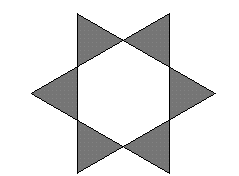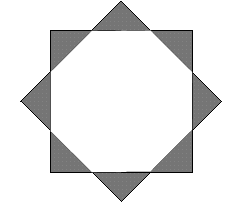#### You may also like### Golden Thoughts

Rectangle PQRS has X and Y on the edges. Triangles PQY, YRX and XSP have equal areas. Prove X and Y divide the sides of PQRS in the golden ratio.### At a Glance

The area of a regular pentagon looks about twice as a big as the pentangle star drawn within it. Is it?### Contact

A circular plate rolls in contact with the sides of a rectangular tray. How much of its circumference comes into contact with the sides of the tray when it rolls around one circuit?

# Star Gazing

##### Age 14 to 16 Challenge Level:

Ned, who has just left Christ Church Cathedral School in Oxford, sent a nice solution saying that: The Star Gazing problem is easy. The 6-point star is made up of 6 equilateral triangles, and so is the hexagon inside it, so the ratio of the points' to the inside' is 1:1''.Ned then found the area of the octagon by dividing it up (a square, 4 rectangles and 4 triangles) and used Pythagoras theorem to find the lengths needed and finally found the ratio of the areas of the points' to the octagon inside' to be 1: (1 + $\sqrt 2$).The second part is most easily done by taking the shorter sides of the triangles which make up the star to have length 1 unit, so that the area of each triangle is 1/2 and the total area of the `points' is 4 square units . The hypotenuse of each triangle has length$\sqrt 2$ (by Pythagoras Theorem) which gives the lengths of the edges of the octagon. To find the area of the octagon take the square and chop off four triangles at the corners.

The length of the side of the square is (2 + $\sqrt 2$)

the area of the octagon = (2 + $\sqrt 2)^2$ - 2

which simplifies to 4 + 4$\sqrt 2$.

Finally the ratio of areas is 4 : (4 + 4$\sqrt 2$) which simplifies to 1 : (1 + $\sqrt 2$ ).